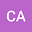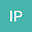••Measuring bedload transport rates usually involves measuring the flux of sediment or collecting sediment during a certain interval of time $\Delta t$. Because bedload transport rates exhibit significant non-Gaussian fluctuations, their time-averaged rates depend a great deal on $\Delta t$. We began by exploring this issue theoretically within the framework of Markov processes. We defined the bedload transport rate either as the particle flux through a control surface or as a quantity related to the number of moving particles and their velocities in a control volume. These quantities were double-averaged, that is, we calculated their ensemble and time averages. Both definitions led to the same expression for the double-averaged mean rate and to the same scaling for the variance’s dependence on the length of the sampling period $\Delta t$. These findings led us to propose a protocol for measuring double-averaged transport rates. We subsequently ran an experiment in a narrow flume using steady-state conditions (constant water discharge and sediment feed rates). Using high-speed cameras in different places, we measured the time variations in the particle flux, the number of moving particles, and their velocities. The data agreed well with the previously defined theoretical relationships. We later applied our experimental protocol to other flow conditions (a long laboratory flume and a gravel-bed river) to show its potential across various contexts.